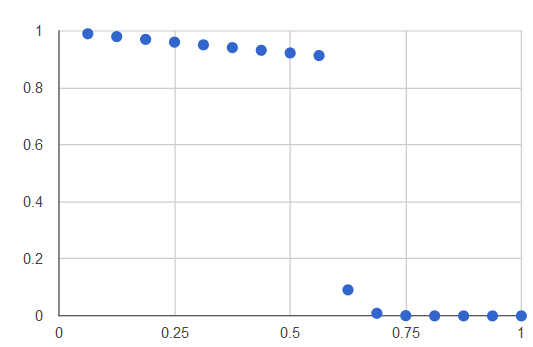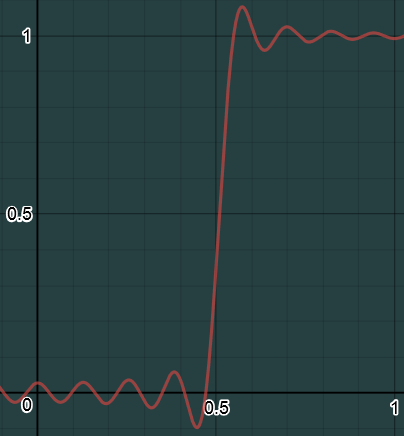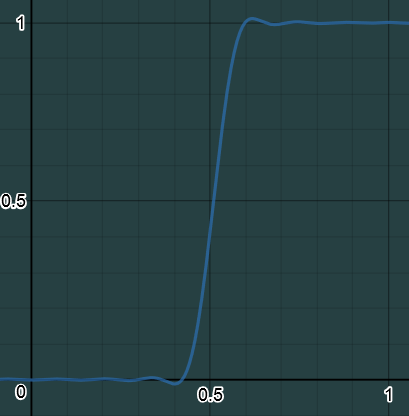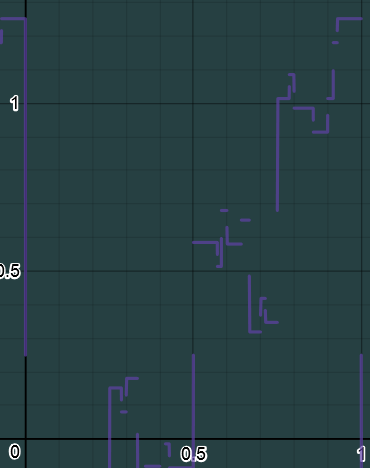## Monday, December 19, 2016

### Fourier series based OIT and why it won't work

I've been doing some research in to using Fourier series or other types of function approximation to do order independent transparency rendering.  While it doesn't look like it can be turned in to a workable solution maybe I can save someone else some time and teach you something about frequency decomposition and function approximation along the way.

## The OIT holy grail

When graphics programmers dream of an OIT solution there are a number of properties that come to mind:

• Bounded memory requirements
• Single geometry pass
• Low performance impact
• No obvious artifacts
• No presorting
• No special hardware requirements
• Works well across the entire depth range
• Allows rendering of opaque elements at the same time
Unfortunately there isn't a solution out there right now that has all these properties. The most popular solution k-buffers and their derivatives have bounded memory requirements, but they can still be quite high and require presorting to avoid artifacts. Solutions like Phenomological OIT provide some good results, but doesn't allow opaque objects to be in the same pass and doesn't give similar results for mostly opaque objects blocking other objects. So it seemed to me that there had to be another path out there.

## Simplifying the problem

When rendering transparent objects they key piece of information we need is how obscured the current fragments contribution to the final pixel (i.e. a multiplier for our color based on how obscured it is). This is commonly referred to as the visibility function. So all OIT solutions fall in to a general pattern of a first pass to generate the visibility function, then a second pass to apply the visibility function to the color data for each visible object in the pixel. If you want to reduce memory requirements you can skip storing the color data for each fragment and instead do a second geometry pass to get access to that information again. While doing a second geometry pass isn't really a great solution, it does allow us to ignore the approximation and compression of color data and focus purely on the approximation of the visibility function. Which makes the path forward look something like this:
1. Render geometry to gather visibility data
2. ???
3. Render second geometry pass and apply visibility function.
4. Profit

## Approximating visibility

Before we talk about the methods of approximation let's look at what a typical visibility function actually looks like.Y is visibility. X is depth.

This shows a bunch of mostly transparent objects and a single almost opaque object.

The million dollar question is how we can generate such a graph that is accurate on both axes and do it one piece at a time so that we can run on DX11 class hardware. What makes this really really hard is that second part: one piece at a time. Since we don't really know about the other parts of the graph that means the approximation can't have a lot of noise in areas away from our sample point because it might affect the values of other sample points and we also have to get a very accurate values around our sample points because they might be very close to another sample point and we don't want inaccurate data when to objects get close together.

My first method was to do this with a combination of square waves (I tried some other methods later, and I'll explain why they failed in a later section). So for each fragment I would generate an approximation of the square wave then add it to the other approximations for that fragment. This of course requires that you can make alpha blending which is normally multiplicative work by adding instead. I came up with a quick formula for that which kind of work: val = 1 - (1.5 * (alpha*2)/(alpha*2+1)).

It's also worth mentioning that a very similar method was published in this paper on Fourier Opacity Mapping for Shadowing. They have a better method for making things additive instead of multiplicative. They also use a sloped function instead of a square wave because they care more about sampling away from the points then at them.

### Taylor Series

The first thing that comes to mind when I hear "function approximation" is the Taylor series. Taylor series work by creating a polynomial that shares the same n-derivatives as the target function at a certain point (i.e. at point X the 0th to nth derivative will be the same value as the target function). This means that to get a good approximation from a Taylor series the function must be continuous at the point, and we have to not really care too much about values away from the sample point. Now lets try to apply that to a square wave. First off square waves have a large discontinuity right at the point we care about, and we also need the values away from that point to be accurate so that we don't add noise to the other sample points. So Taylor series are right out.

### Fourier Series

Fourier series are a better choice because they allow discontinuities for the function. Fourier series work by decomposing the function in to the frequency domain and storing only a fixed number of frequency coefficients. One big problem here is that a square wave has is composed of an infinite number of frequencies, so we'll have to deal with noise of some kind. So the Fourier series approximation for a square wave ends up looking something like this:Cosine Fourier series approximation for square wave of magnitude 1 at x = .51, with n=15

There are three big problems with this graph. The first problem is that the value at our sample point (in the graph x=.51) is not actually the value we want. The second is the oscillating noise. We need to get rid of that noise so that we're not adding too much noise to other sampling points. And the third problem is that we have this big wind up and overshoot around our sample point.

First lets look at the value at our sample point. This one is actually pretty easy to deal with. It turns out that at discontinuities Fourier series converge to the half way point between the two converging limits. Since we already have additive values from the graph and we know what our alpha is when we sample the point we can just add half of our alpha value to the sample point to get the proper value.

Next lets look at the noise problem. There are standard methods for dealing with this noise which is known as the Gibbs phenomenon. This is the same phenomenon that causes ringing in spherical harmonics which are basically Fourier series on a sphere. All the common methods for dealing with this problem boil down to one thing: blurring. The method I settled on was the sigma approximation which is described here. Which turns the above graph in to this:Sigma smoothed cosine Fourier series for n = 15 d = .51 a = 1

This has actually taken care of the noise problem fairly well, but has made the wind up and overshoot around our sample point stretch longer across X.

Which leads in to the third problem. How do we deal with those wind ups and overshoots? After much research my conclusion is: I dunno. But just for fun let's go through a quick list of all the stuff I've tried so far.

•  Try using a square wave basis instead of a sine wave basis. You can actually use any periodic functions that are orthonormal for a Fourier series. I tried square waves of increasing frequencies. But it took a ton of frequencies to get to a graph the looked even remotely close and even then the noise level was off the charts. For fun here's a n=15 graph for square wave reconstruction at d=.51.• Look at the derivatives around the sample point. The idea here was I could look at it then estimate how far along the transition I was for the other points. This was actually pretty good at finding out how much we were going to transition (i.e. how much alpha was being added). The problem here is that the distance between two objects can be very very small in things like particle systems so it tended to break down in those cases. And in cases where there were many objects close together I found no method of determining how much alpha was before my sample point and how much was after it.
• Just use the value you get. Maybe I can just ignore all that? No, because now particles that are behind an object in depth start to obscure the objects in front of them.
• Smooth it with Lancosz or some other function instead of sigma. Nope, they all just blur it more or less.
• Wavelets? Aren't easily addable like Fourier coefficients. Are used mostly for discrete values where you have all the samples at one time.
• Use a Fourier cosine series to get better approximations in the same space. All the graphs here are actually just using a Fourier cosine series since it does approximate it better than a sine/cosine series if we're willing to make the function odd, which we can because we don't care about the function before 0. But it still doesn't get us close with a reasonable number of terms like n=15.
• Try using a b-spline or FIR basis or something not based on frequency decomposition. I haven't actually tried this one yet, but I think it will have the same problem of overlapping values as Fourier does.

## Conclusion

So that is where my research has ended. Unless the problem above can be solved I don't think this could be usable for a production game renderer. Maybe there is some great solution out there to reconstruct this signal properly, but I've run out of ideas for now and have tabled the research. If you have any great insights here you can contact me on twitter. Thanks for reading!